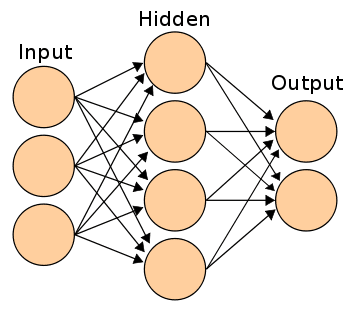Submit a blog to KDnuggets -- Top Blogs Win A Reward

Topics: AI | Data Science | Data Visualization | Deep Learning | Machine Learning | NLP | Python | R | Statistics

KDnuggets Home » News » 2016 » Apr » Tutorials, Overviews » Holding Your Hand Like a Small Child Through a Neural Network – Part 2 ( 16:n15 )

# Holding Your Hand Like a Small Child Through a Neural Network – Part 2

The second of 2 posts expanding upon a now-classic neural network blog post and demonstration, guiding the reader through the workings of a simple neural network.

By Paul Singman, Freelance Data Scientist.For Part I of this riveting series, click here.

In Part I, we went through each calculation by hand of a forward and backward pass through a simple single-layer neural network.

To start Part II, we’re going to do the same for the second pass through. My hope is after doing this a second time trends will emerge and we will be able to understand how the network’s weights end up where they do by 100,000th pass.

Since the first pass was called iteration #0, we begin with iteration #1:

```
---------ITERATION #1-------------
inputs:
[[0 0 1]
[0 1 1]
[1 0 1]
[1 1 1]]

weights:
[[ 0.67423821]
[-0.33473064]
[-0.40469539]]

dot product results:
[[-0.40469539]
[-0.73942603]
[ 0.26954282]
[-0.06518782]]

l1 probability predictions (sigmoid):
[[ 0.40018475]
[ 0.32312967]
[ 0.56698066]
[ 0.48370881]]

```

Compared to the first pass, the first weight is larger and the second two weights got smaller. We’ll see if these updated weights cause less error in our predictions (Spoiler: They will).

Although you should be able to do dot products in your sleep at this point since you followed along so closely with Part I of the series, I’ll walk us through the dot product again:

```
(0 * .674) + (0 * -.335) + (1 * -.404) = -.4047
(0 * .674) + (1 * -.335) + (1 * -.404) = -.7394
(1 * .674) + (0 * -.335) + (1 * -.404) = .2695
(1 * .674) + (1 * -.335) + (1 * -.404) = -.0652

```

Great. Now we run the results through the sigmoid function to generate probability predictions (shown as “l1 probability predictions (sigmoid) above).

For nostalgia’s sake, here were our predictions from the previous pass:

```
OLD l1 probability predictions (sigmoid):
[[ 0.36672394]
[ 0.27408027]
[ 0.46173529]
[ 0.35868411]]

```

If you compare the old predictions with the new ones, you’ll notice that they simply all went up, meaning the model thinks they are more likely to be ones than before.

In terms of error, it hasn’t improved much from the last run.

```
OLD l1_error:
[[-0.36672394]
[-0.27408027]
[ 0.53826471]
[ 0.64131589]]

```

```
NEW l1_error:
[[-0.40018475]
[-0.32312967]
[ 0.43301934]
[ 0.51629119]]

```

Calculating the sum of the absolute value of the four errors, it did decrease from 1.82 to 1.67. So there was improvement!

Unlike in Part I, I’m not going to dive into the details of how taking the derivative of the sigmoid at the spot of the probability prediction, multiplying the result by the errors, and then taking the dot product of the result with the inputs leads to updating the weights in a way that will reduce prediction error… but instead just skip to the updated weights:

```
pre-update weights:
[[ 0.67423821]
[-0.33473064]
[-0.40469539]]

post-update weights:
[[ 0.90948611]
[-0.27646878]
[-0.33618051]]

```

## Top Stories Past 30 Days

Most PopularGet KDnuggets, a leading newsletter on AI, Data Science, and Machine Learning

By subscribing you accept KDnuggets Privacy Policy